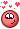[[Template core/front/profile/profileHeader is throwing an error. This theme may be out of date. Run the support tool in the AdminCP to restore the default theme.]]

## Posts posted by Librarian

1. ### How can I use the hip name as the flipbook output name?

@Masoud Try This ,maybe you gonna need to install some Codec's .
Sop and Top level.

2. ### Magic Trail effect

@trinityNoob Then Just use PopCurve Force with PopFluid , you have ex in Documentation

3. ### Procedural Pen Writing up Effect

@popsicle Just use SINGLE LINE Fonts for engraving CNC embossing  ...

4. ### Worley noise (chebyshev) not showing up as expected

change box to polygon-mesh not Mesh ..Definitely this is  the Case in your Ex @archz2,
use periodic Worley Noise, look like its a BUG.hm

•1
5. ### Worley noise (chebyshev) not showing up as expected

4 points in the scatter param I think ..just change

•1
6. ### Tutorial HIP Library

•4
7. ### line to 2d mesh vex?

```vector pos(float u,t){
vector p = set(0,u,0);
p+= u *0.2*curlnoise(p+0.1*t);
return p;

}

int npt = 30;
for (int i;i<npt;i++){

float u = i/float(npt-1);
vector p= pos(u,@Time);
vector next_p = pos(u+0.1,@Time);
vector up = normalize(next_p-p);
vector4 ori = dihedral({0,1,0},up);
int pt = addpoint(0,p);
setpointattrib(0,"orient",pt,ori);
matrix3 r =qconvert(ori);
vector v = set(r.xx,r.xy,r.xz);
vector side = set(r.zx,r.zy,r.zz);
setpointattrib(0,"v",pt,v);
setpointattrib(0,"side",pt,side);
}```
```int pts0[];
int pts1[];

for(int i;i<i@numpt;i++){

vector p = point(0,"P",i);
vector dir = point(0,"side",i);
dir = normalize(dir);
float u = float(i)/(@numpt-1);
u = chramp("ramp",u);
vector p0= p+dir*u;
vector p1= p-dir *u;
int pt0 = addpoint(0,p0);
append(pts0,pt0);
append(pts1,pt1);

}

for (int i;i<@numpt-1;i++){

}```

use Sweep Node Best

8. ### Tutorial HIP Library

human error11. ### [SOLVED]Vex? Issue?

```int count = chi('count');
float seed = chf('seed');
int iarr[] = array();
for (int i = 0;i<@numpt;i++){
iarr[i]=i;
}

function void shuffle( int a[];float seed
float b[] = {};
for(int i = 0;i<len(a);i++){
push(b,rand(i+seed));
}
int c[] = argsort(b);
a = reorder(a,c);
}
shuffle(iarr,seed);
i[]@arr = iarr;
i@variant = iarr[@ptnum]%count;

//i@variant = floor(rand(@ptnum)*count);```

function void shuffle( int a[];float seed
this Line its Wrong total Stop
Solved

"function void shuffle(int a[];float seed ){"

12. ### Rotating each item on its own individual axis?

•1
13. ### Rotating each item on its own individual axis?

@archz2 create att rot than wrangle

`p@orient = eulertoquaternion(radians(v@rot), XFORM_XYZ);`

•1
14. ### glaze dripping effect on pottery

hm maybe to use shallow-water Solver then you can use those attributes on texture and make displacement its fast  you have 2 ex in .
C:/Program Files/Side Effects Software/Houdini 19.5.303/houdini/help/examples/nodes/

•1
15. ### Particle Morph on animated geometry

hm @popsicle No problem here to use Adam Swab Tutos on 19.5.337 it works ..obsolute or nothe just use Vex on that ex .
You have also nice ex on you tube, particles morph using Vellum Glue

16. ### Tutorial HIP Library

•5
×